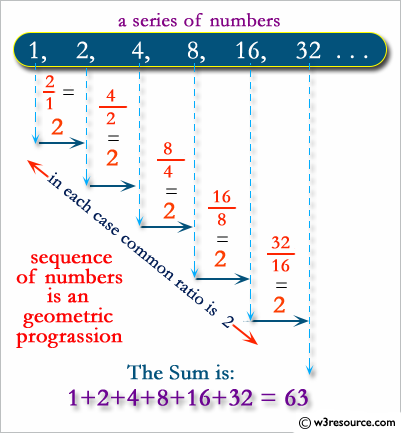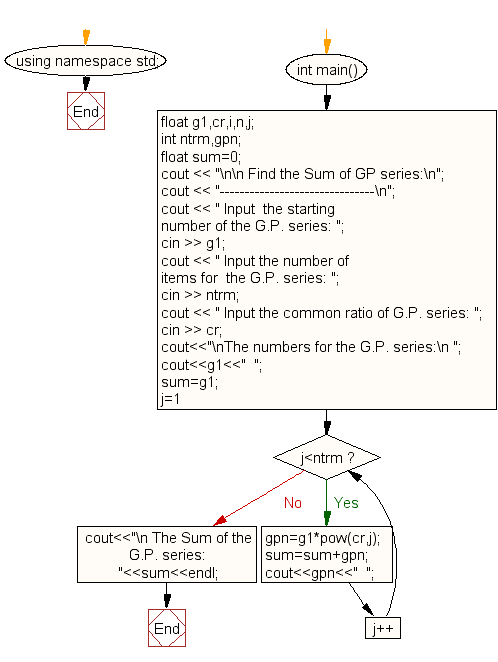﻿ C++ Exercises: Find the Sum of GP series - w3resource# C++ Exercises: Find the Sum of GP series

## C++ For Loop: Exercise-32 with Solution

Write a program in C++ to find the Sum of GP series.

Pictorial Presentation:Sample Solution:-

C++ Code :

``````#include <iostream>
#include <math.h>
using namespace std;

int main()
{
float g1,cr,i,n,j;
int ntrm,gpn;
float sum=0;
cout << "\n\n Find the Sum of GP series:\n";
cout << "-------------------------------\n";
cout << " Input  the starting number of the G.P. series: ";
cin >> g1;
cout << " Input the number of items for  the G.P. series: ";
cin >> ntrm;
cout << " Input the common ratio of G.P. series: ";
cin >> cr;
/*-------- generate G.P. series ---------------*/
cout<<"\nThe numbers for the G.P. series:\n ";
cout<<g1<<"  ";
sum=g1;
for(j=1;j<ntrm;j++)
{
gpn=g1*pow(cr,j);
sum=sum+gpn;
cout<<gpn<<"  ";
}
/*-------- End of G.P. series generate ---------------*/
cout<<"\n The Sum of the G.P. series:  "<<sum<<endl;
}
``````

Sample Output:

``` Find the Sum of GP series:
-------------------------------
Input  the starting number of the G.P. series: 3
Input the number of items for  the G.P. series: 5
Input the common ratio of G.P. series: 2

The numbers for the G.P. series:
3  6  12  24  48
The Sum of the G.P. series:  93
```

Flowchart:C++ Code Editor:

Contribute your code and comments through Disqus.

What is the difficulty level of this exercise?

﻿